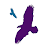## Pages

### Generating Fibonacci numbers in Delphi: Recursive and iterative algorithms

In this post, I want to implement a function that returns the Nth Fibonacci number. Initially, I will provide a recursive implementation that derives directly from the Fibonacci sequence definition. Afterwards, I will recode the same function using an iterative approach.

Why do I want to do (share) such a thing? Well, firstly for fun :-) and secondly, because I was asked to do something similar in one phone screen interview. Really? Yep, I was asked to code a function to return the factorial of a number and then, I had to read it over the phone. I implemented the recursive algorithm. At this point, I was asked why I decided to use recursion as opposed to iteration. My answer was that I find the recursive implementation easier (and cleaner) to write. The interviewer finally inquired me about the iterative implementation…

This motivated me to resolve similar programming tasks (recursively and iteratively) just as a training exercise.

Well, enough with that blah, blah, blah.

Taken from Wikipedia:

The Fibonacci numbers form a sequence of integers, mathematically defined by

F(0)=0; F(1)=1; F(n) = F(n - 1) + F(n - 2) for n > 1.

This results in the following sequence of numbers:

0, 1, 1, 2, 3, 5, 8, 13, 21, 34, 55, 89, 144, 233, 377, 610, 987, ...

This simply means that by definition the first Fibonacci number is 0, the second number is 1 and the rest of the Fibonacci numbers are calculated by adding the two previous numbers in the sequence.

Translating that into Delphi code:

`function Fibonacci(aNumber: Integer): Integer;`
` begin`
`   if aNumber < 0 then`
`     raise Exception.Create('The Fibonacci sequence is not defined for negative integers.');`
` `
`   case aNumber of`
`   0: Result:= 0;`
`   1: Result:= 1;`
`   else`
`     Result:= Fibonacci(aNumber - 1) + Fibonacci(aNumber - 2);`
`   end;`
` end;`
` `

The function above is the recursive implementation, which in my opinion fits naturally. Now, the iterative implementation might not be as cleaner as that:

`function Fibonacci(aNumber: Integer): Integer;`
` var`
`   I,`
`   N_1,`
`   N_2,`
`   N: Integer;`
` begin`
`   if aNumber < 0 then`
`     raise Exception.Create('The Fibonacci sequence is not defined for negative integers.');`
` `
`   case aNumber of`
`     0: Result:= 0;`
`     1: Result:= 1;`
`   else`
`     begin`
`       N_1:= 0;`
`       N_2:= 1;`
`       for I:=2 to aNumber do`
`       begin`
`         N:= N_1 + N_2;`
`         N_1:= N_2;`
`         N_2:= N;`
`       end;`
`       Result:= N;`
`     end;`
`   end;`
` end;`
` `

Finally, if you want to produce the first 21 Fibonacci numbers try this out:

`program Project2;`
` `
` {\$APPTYPE CONSOLE}`
` `
` {\$R *.res}`
` `
` uses`
`   System.SysUtils;`
` `
` var`
`   I: Integer;`
` `
` function Fibonacci(aNumber: Integer): Integer;`
` begin`
`   {Your implementation goes here}`
` end;`
` `
` begin`
`   for I:=0 to 20 do`
`     Writeln(Fibonacci(I));`
`   Readln;`
` end.`
` `

Hopefully you are not bored to death :-)

1.If you enjoy these sort of programming diversions then you will almost certainly gain a lot of entertainment value (and perhaps intellectual challenge) from Project Euler...

http://projecteuler.net

2.Thank you Jolyon! I really enjoy these kind of challenges: Math has always been my love :-D

3.You might also be interested in contributing to the RosettaCode site.

http://rosettacode.org/wiki/Category:Delphi

4.Hi Bruce,

Actually, I have been contributing. I wrote a few tasks already. I am keeping track of them in one of my posts: http://www.yanniel.info/2011/08/contribute-to-delphi-at-rosettacode.html

Now that you mention is been a while since the last time I visited Rosetta Code.

5.Two more approaches, just for fun of it: http://www.thedelphigeek.com/2011/12/fibonacci-numbers-weird-way.html

6.7.Good evening! How do I test the recursive function?
I put a button, an Edit (to enter the value) and a TMemo. I called the function by passing the value of Edit and in the memo I passed the Result to see what was happening ... but it is not returning correctly. For example, digit 5 ​​and it does not bring me correctly 0,1,1,2,3,5. Did I get it wrong?!?